# Choose Your Best Bar Exam Prep Option with a Decision Matrix

Still having a hard time deciding which bar prep program option to decide on?  Check out this decision matrix we made for you to use.

We make decisions using both our logic and intuition.  The matrix will break down the logical side in plain numbers and figures for you.  But, use your intuition when deciding as well.  It knows a lot more about you and the world than you think.  Here’s what it looks like: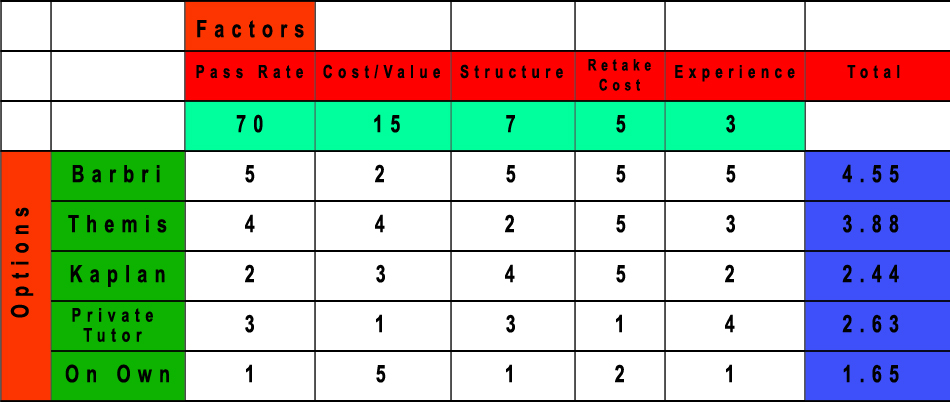How the matrix works:

### Step 1:  Write out the question you want to decide on

In our case, the question is, “Which bar prep program should I take?”

### Step 2: Write out each of the bar prep OPTIONS you are deciding between

We selected Barbri, Themis, Kaplan, Private tutor, and self study.  However, you may find other programs or options you want to consider.### Step 3: Write down all the FACTORS that are important to you

We wrote down the factors we felt were important in deciding which bar prep program we would want to take:  1) pass rate, 2) cost, 3) structure, 4) cost if have to retake, and 5) experience of the program.  You may have a different list depending on your own circumstances, so feel free to adjust as you see fit.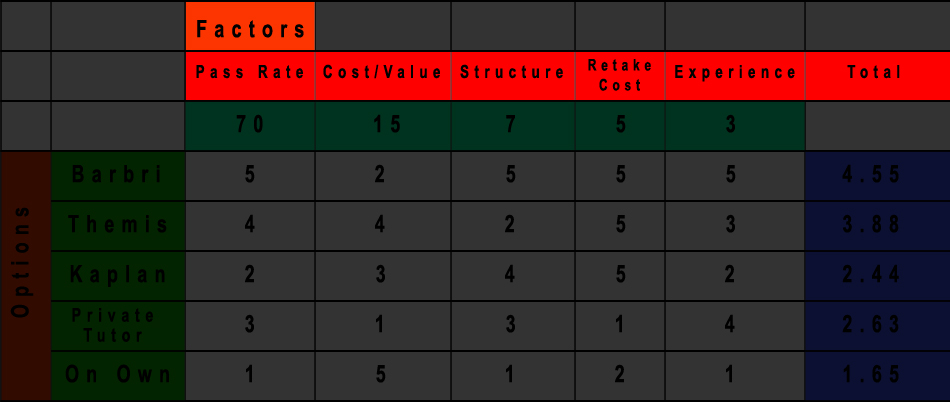### Step 4:  Give each factor a number based on its importance to you

Now, we weigh each factor based on how important it is to us, giving the most points to the most important factors.  The total points should add up to 100.  We gave 1) pass rate a high 70 points because this was the most important thing to us by far.  The 2) cost was second with 15 points, and so on.  If you don’t agree with the numbers, you can always pick your own.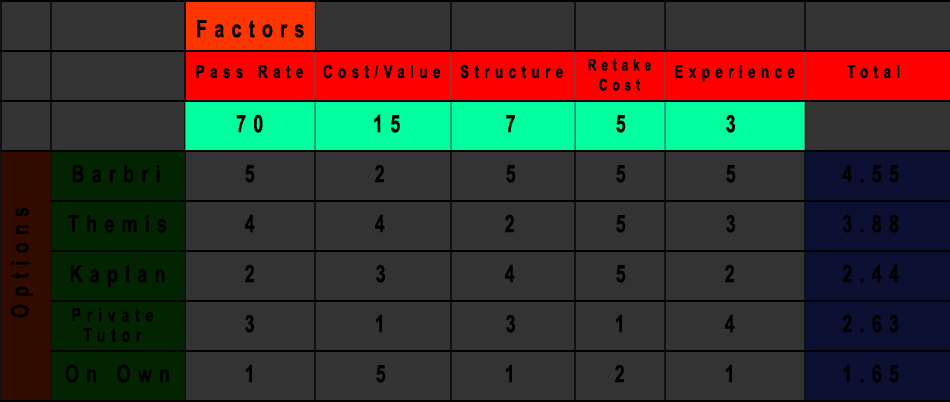### Step 5:  Rank each program for each category

Next, we go through each FACTOR and rank each option in that category.  We have 5 program options; therefore, each program OPTION should get a number between 1 and 5.  We gave a ‘5’ to the best program in that factor category and a ‘1’ to the worst.  For example, Themis got a ‘4’ on the cost factor because it has the second cheapest cost, next to self study.  Barbri got a ‘5’ on the pass rate because it supposedly has the best pass rate.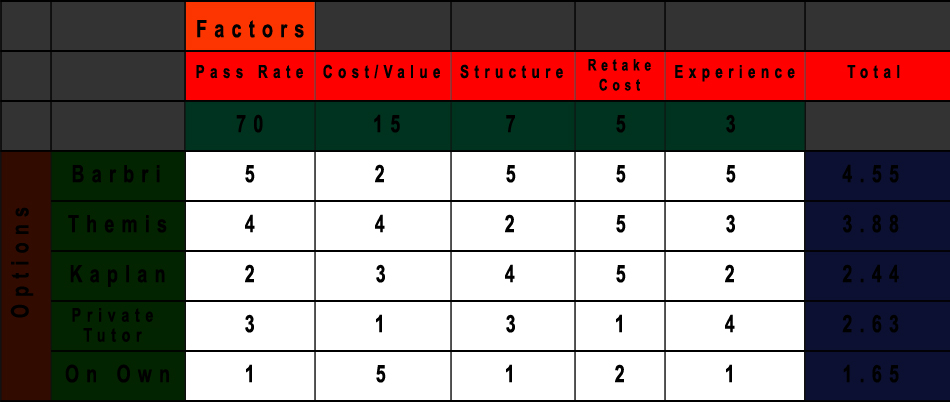### Step 6:  Multiply and Add to get the totals

Then we go down the horizontal line for each OPTION and multiply the 1 to 5 ranking we gave by the weight of that factor, adding up all the factor numbers, then dividing by 100 to get our final score for that option.  For Barbri, we went (5*70 + 2* 15 + 5*7 + 5*5 + 5*3)/100 = 4.55.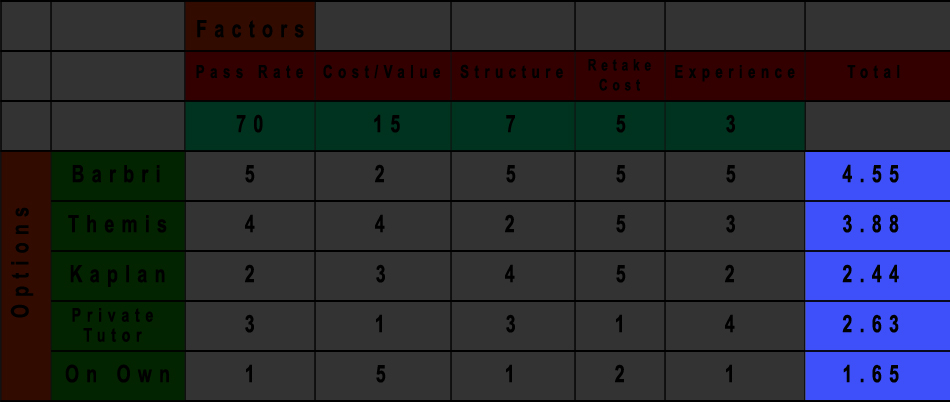### Step 7: Decide

Once you do that for all the options, you now can see directly in front of you how well each option did based on the criteria you gave it.  Then you can make a more informed decision!

We made the spreadsheet using excel in a few, short minutes.  If you want to change the weight of the factors, add or subtract options, or just want the excel spreadsheet, email us at dustin@ipassedmybarexam.com and we’ll send the sheet over to you.  Don’t worry, we won’t sell your email address to Barbri!

Best of luck on making a decision and passing your bar exam!

“This name appears on the pass list”

##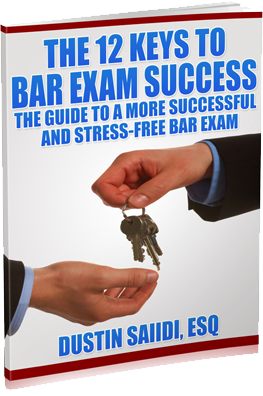Yes, I want My Free Guide!